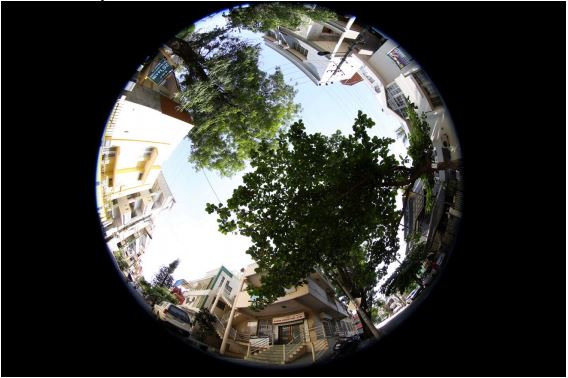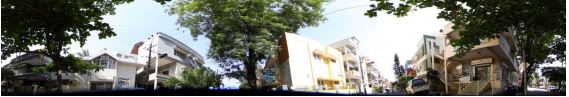## DEWARPING & PANORAMA GENERATION FROM FISHEYE IMAGES

### Overview - Panorama generation from the periphery of a fisheye

#### Input and output

This uses an input like:To generate an output like:This is the view around from the crossroad at which the Atria BLR office is located.

### Libraries used

1. Simple CV a python wrapper on OpenCV, facilitating the input and output of data from various modalities into OpenCV
2. OpenCV with Python bindings allow Python interfaces for OpenCV functions
3. NumPy for math routines used in Python script

### Algorithms

The main modules required for Panorama generation from single shot taken with a fisheye lens, where the principal axis is vertical, are:

• Identification of the center, inner radius and outer radius of the fisheye image "donut"
• Building the map: warped -> rectilinear, which is a mapping of the location of the individual pixels of the fisheye image that form the rectilinear panorama image.
• Applying the above map to construct the panorama image from pixels of the fisheye image.

### Identification of the center, inner radius and outer radius of the fisheye image

This is manually determined here, by clicking on the approximate center point [Cx Cy], and subsequently selecting 2 points to denote the inner and outer radii of the fisheye image [R1x R1y], [R2x R2y]. These points are used to determine the inner and outer radii as R1 and R2
R1 = R1x-Cx
R2 = R2x-Cx
These parameters, C, R1 and R2 determine the coordinate system that is, in turn, used to define the mapping in the next section.

The fisheye image resembles a donut, because the region defined by the inner radius, centered at the center, is not processed. Only the annular region from the inner to the outer radius is used; hence donut. The central region is not used to avoid the extreme distortion that would be caused if it were.

### Building the map

First, source and destination image sizes are determined as [Ws Hs] and [Wd Hd]
Wd = 2.0*((R2+R1)/2)*pi
Hd = (R2-R1)
Ws = img.width # img is the source fisheye image
Hs = img.height

Then, the following transformation is used to map which source pixels the destination pixels originate from:
r = (float(yD)/float(Hd))*(R2-R1)+R1
theta = (float(xD)/float(Wd))*2.0*np.pi
xS = Cx+r*np.sin(theta)
yS = Cy+r*np.cos(theta)

Here, [r theta] coordinates in the fisheye image, for each of the destination pixels [xD yD] are found using the parameters determined earlier R1 and R2, with the origin at C. Then, the coordinate origin is shifted to the origin of the image to get [xS yS].

The arrays xmap and ymap hold the source coordinates from which destination coordinate [xD yD] originates:
xS = xmap[xD yD]
yS = ymap[xD yD]

### Applying the map

The map generated in the previous section is applied to the fisheye image (using bilinear interpolation to construct values for integer pixel values, where the map contains floating values). OpenCV function 'remap' is used to do this.
output = cv2.remap(img,xmap,ymap,cv2.INTER_LINEAR)

The function 'remap' transforms the source image using the specified map:
dst(xD, yD) = src(xmap(xD,yD),ymap(xD,yD))

where, values of pixels with non-integer coordinates are computed using one of available interpolation methods.

xmap and ymap can be encoded as separate floating-point maps in map1 and map2 respectively, or interleaved floating-point maps of (x,y) in map1 , or fixed-point maps created by using cv2.convertMaps(). The reason you might want to convert from floating to fixed-point representations of a map is that they can yield much faster (~2x) remapping operations. In the converted case, map1 contains pairs (cv2.Floor(x),cv2.Floor(y)) and map2 contains indices in a table of interpolation coefficients.

This function cannot operate in-place.

### Areas of improvement/optimization

• Identification of the center could be automated using circular Hough transform, because it is inaccurately done manually, resulting in the black patch towards the bottom of the image in some parts.
• The panorama image looks a little washed out because of the resampling using bilinear interpolation. There are other interpolation kernels like bicubic and Lanczos4, which might yield better quality at the price of computational cost. An alternative would be to use hardware accelerators to offload these heavy computational tasks to, so as not to sacrifice quality or real-time performance.
• An adjustment for the angular position of the fisheye image at which the cut happens could be provided to the user, so that s/he can choose where the panorama splitting should happen, thereby saving objects of interest from ending up split at two ends of the panorama.
• The image needs perspective correction, so that the objects to appear as having been imaged from a low altitude.